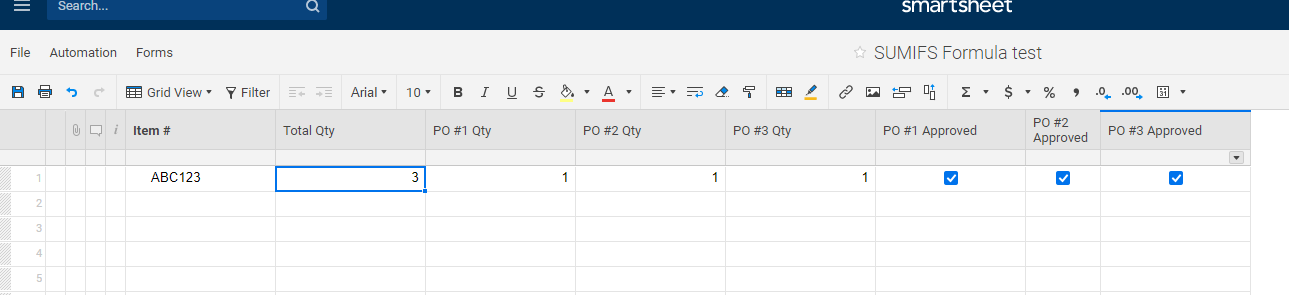# SUMIFS formula question

I’m working on a SUMIFS formula to add/sum numbers in 2 or more separate cells based on a checkbox input in 2 or more other cells. I’m able to do so only if the number columns and the checkbox columns are next to another.

Is there a way to do so even when the columns are NOT next to the other?

Here are snapshots:The formula used on the “Total Qty” column is  =SUMIFS([PO #1 Qty]1:[PO #3 Qty]1, [PO #1 Approved]1:[PO #3 Approved]1, =1)

• Sorry, here's the snapshot• It is possible, but you would need to find a way to specify through criteria which columns to sum. Can you provide an example of the layout you WANT to use?

• Yes i have a similar question- I want to SUM 2 cells only if a specific check box is checked .

In other words IF(Check 14) is checked yes- THEN i wish to ADD (SFR VAL13)and (SFR VAL14)

see screen shot- is this possible- My formula is not working but its as close as I can get.

• Try something like

=IF(CHECK14 = 1, SUM([SFR VAL]13, [SFR VAL]14))

## Help Article Resources

Want to practice working with formulas directly in Smartsheet?

Check out the Formula Handbook template!